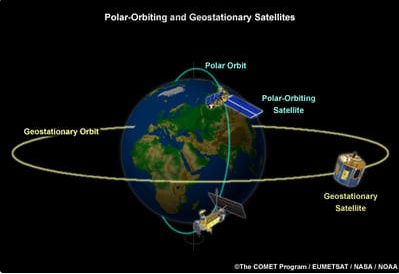# Geostationary and Polar Satellites

#### description

• Geo-Stationary Satellites

#### notes

Geostationary Satellite:-

• Geo means earth and stationary means at rest. This means something which is stationary.

• Satellites orbiting around the Earth in an equatorial plane with time period equal to 24 hours.

• Appear to be stationary with respect to earth. They also rotate around the earth with a time period of 24 hours.

• These satellites can receive telecommunication signals and broadcast them back to a wide area on earth.

• Example: INSAT group of satellites.

Problem:- Calculate the height of a geostationary satellite from the surface of the earth?

Answer: For any geostationary satellite time period

T = 24hours = 24xx60xx60s

= 86400 sec

orbital velocity "v"=(2pi"R")/"T"

Where R= distance of satellite from the earth. it is given as "R" = "R"_E + "h"

"F"_c = "mv"^2/"R"

"F"_G = ("GM"_e"m")/"R"^2

"F"_c = "F"_G

"mv"^2/"R"=("GM"_e"m")/"R"^2

By simplifying,

"v"^2 = "GM"_e/"R"

=(4pi^2"R"^2)/"T"^2="GM"_e/"R"

"R"^3="GM"("T"^2/(4pi^2))

Acceleration due to gravity "g"= "GM"/"R"_E^2

"GM"="gR"_E^2

"R"^3=("gR"_"E"^2"T"^2)/(4pi^2)

therefore "R"= [("gR"_E^2"T"^2)/(4pi^2)]^(1/3)

by putting the values and calculating

R = 42147km

"R"="R"_E + "h"

"h"="R" -"R"_E

=(42147 - 6.37 xx 10^3)

h = 35777km

The height of the geostationary satellite from the surface of the earth is 35777km.

Polar Satellites

• These are low altitude satellites. This means they orbit around the earth at lower heights.

• They orbit around the earth in North-South direction. Whereas the earth is moving from East to West.

• A camera is fixed above this type of satellite so they can view small strips of earth.

• As earth also moves, so at each instance different types of stripes of earth can be viewed.

• Adjacent stripes of the earth are viewed in subsequent orbits.

• They are useful in remote sensing, meteorology and environmental studies of the earth.If you would like to contribute notes or other learning material, please submit them using the button below.

### Shaalaa.com

Geo-Stationary Satellites [00:34:03]
S
0%